Next: 3.2.2 Vertical resolution: probe Up: 3.2 Titan atmospheric descent: Previous: 3.2 Titan atmospheric descent:

### 3.2.1 Probe motion during descent

The motion of the Huygens Probe in Titan's atmosphere is determined by the action of two forces, gravity and wind drag. The acceleration due to wind drag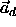is taken from Flury (1986) to be: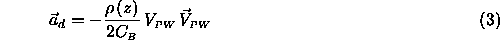where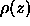is the atmospheric density,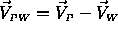is the relative velocity of the Probe with respect to the atmospheric wind, and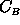is the ballistic coefficient (units: kg/m˛) defined by: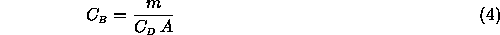with m = probe mass,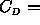drag coefficient, and A = effective probe area to the flow.

The wind velocities in the (x,y,z) directions of a Cartesian coordinate system on Titan's surface are given by:

```
u =	 zonal wind      	 (x-axis: positive toward east)

v =	 meridional wind 	 (y-axis: positive toward north)

w =	 vertical wind   	 (z-axis: positive upwards)

```
The Probe's equation of motion during the Titan descent phase can thus be written: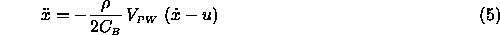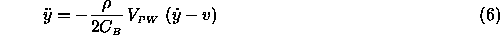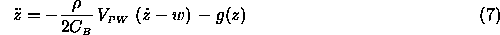where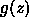is Titan's gravitational acceleration, and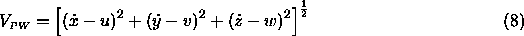The meridional and vertical winds, v and w, are assumed to be considerably weaker than the zonal wind u. In this case, the latitude of the Probe (y component) remains approximately constant.

Knowing the Probe's velocity on Titan, it is not difficult to determine the Doppler shift projected onto the PRL ray path back to the Orbiter: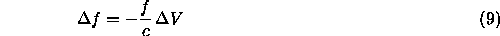where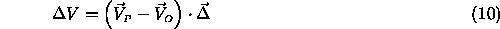with

```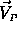= probe velocity w.r.t. Titan center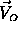= orbiter velocity w.r.t Titan center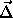= unit vector pointing from Orbiter to Probe

```
The vectordefines the line-of-sight of the PRL.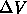is negative during the Titan descent, so that the received frequency is increased (blue shifted). The PRL Doppler shift (9) is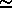37.6 kHz for the nominal orbiter starting approach velocity of -5.53 km/s. The velocity of the Probe projected onto the line-of-sight can be written: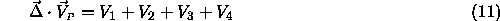where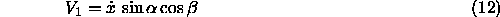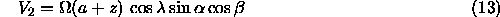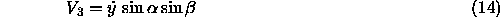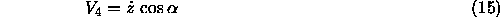Furthermore, we define the projection of the orbiter velocity onto the line-of-sight by: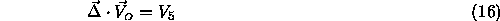The angles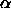and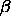define the direction from the Probe to the Orbiter in a local Cartesian coordinate system on the Probe oriented along a natural Titan coordinate grid (x-axis positive toward east; y-axis positive toward north; z-axis positive upward). The angle, basically the zenith angle of the Orbiter as seen from the Probe (Ott 1991), is also sometimes designated as the "probe aspect angle" (PAA). The azimuthal angleof the Probe-to-Orbiter line-of-sight is labeled the "line-of-sight azimuth" (LOSA) by some authors (Atkinson 1989, Pollack et al. 1992).

The term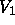in (12), where the mean value of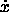u, the drift velocity due to zonal winds, is the quantity to be determined by DWE. This velocity is co-aligned with the contribution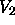in (13) due to Titan rotation. It is assumed for simulation purposes that the rotation is synchronous with the Titan orbital period (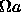11.7 m/s). As noted in the previous section, the relative importance of, and thus the quality of the reconstructed wind profile, is strongly dependent on the values ofand.

The term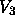in (14), arising from meridional drift, should be small since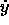is not expected to be important. The term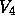in (15) contains the vertical descent velocity. This quantity can be obtained to a high degree of accuracy either from the range rates deduced from the Probe's proximity sensor data, or using measurements of temperature T and pressure P from the Huygens Atmospheric Structure Instrument (HASI). In the latter case, based on the assumption of hydrostatic equilibrium and ideal gas behavior, the descent velocity is determined from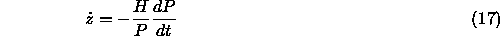where variations in H =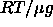, the atmospheric scale height, are assumed to be negligible over the altitude range of the Huygens descent. The final error associated with the determination of the reconstructed probe descent velocity is estimated to be of the order of 1%.

Knowing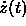from the proximity sensor or from HASI measurements, it is possible to reconstruct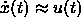from the Doppler shift of the PRL (9). Using in situ measurements of the density, we can then extract the exact height profile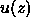from the motion equations (5) and (7). Comparitive wind measurements are expected near the surface from two independent sources on board the Huygens Probe:

• Proximity sensor measurements utilizing pendulum swing motion, or
• Inference of horizontal motion from the Descent Imager & Spectral Radiometer (DISR).
This would provide verification of the DWE height profile for the last few data points prior to touchdown where horizontal winds are likely to be weak. If the Probe survives the surface impact and continues to transmit, further DWE measurements might provide a frequency reference for zero wind. The post-impact data are not necessarily a reliable calibration, however, because the probe oscillator may execute shock-induced frequency shifting at impact.

In order to provide a rough estimate of the Doppler shifts involved, Fig. 4 shows the results of a simplified calculation of the five line-of-sight velocity contributions to the PRL frequency shift,through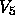. The geometry for the calculations was taken from the nominal Huygens descent profile on Titan (duration: 135 min). The upper and lower panels show the same curves on different ordinate scales.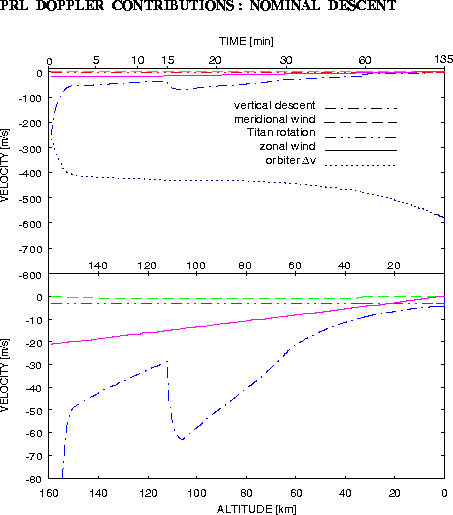Figure 4: Velocity contributions to PRL Doppler shift for the nominal Huygens descent profile.

Only the change in the projected orbiter velocity from its initial value, i.e.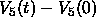, is plotted in Fig. 4. The total projected velocity is plotted for the other contributions, all of which happen to be negative (blue shift in PRL frequency) in this example. The 100% nominal zonal wind model was used for computing the zonal wind contribution. The Titan rotation termis nearly constant during the entire descent. The meridional winds inwere taken to be a constant +1 cm/s (blowing toward north). The change of parachutes at t = 15 min is clearly seen in the curve for, which is the second largest contributer to the changing Doppler shift.

In addition to the derivation of a zonal wind height profile from the large-scale drift, DWE may be able to provide valuable science and navigation services from the small-scale variations in frequency as a 'by-product' of the analysis. Two such possibilities are:

(1)
Knowledge of the zonal wind velocity enables an extremely accurate reconstruction of the Huygens' flight path during descent, and therefore the best possible determination of the impact coordinates on Titan.
(2)
Rotational motion of the Probe during descent will produce a small modulation in the PRL frequency and signal level that will be exploited to derive the spin rate and spin phase of the Probe, thereby effectively serving as a compass in the Titan landscape.

The amplitude of the Doppler modulation in item (2) is proportional to the spin rate. The ultimate accuracy of the Doppler measurement, limited by the clock digitization of the PSA receivers, is 60 mHz. This accuracy translates to an estimate of the spin rate error (1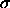) of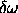=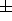0.27/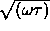rpm, where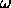is the spin rate in rpm and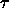is the integration time in minutes. For a representative value of= 1 min, this yields a relative error of 27% just prior to impact, when the probe spin rate is expected to be1 rpm. The relative error is much smaller at higher altitudes where the spin rate is greater (e.g.,0.9% for= 10 rpm,= 1 min). An improvement in this estimate from the Doppler data can be achieved by including the signal level data. Because the radiation pattern of the Probe Transmitter Antenna (PTA) is asymmetric, the Probe's rotation phase can be determined from the phase of the received signal level modulation. The amplitude of PTA's cyclic variation will be ca.2 dB during each rotation.Next: 3.2.2 Vertical resolution: probe Up: 3.2 Titan atmospheric descent: Previous: 3.2 Titan atmospheric descent:

DWE Homepage - For further information contact r.dutta-roy@freenet.de